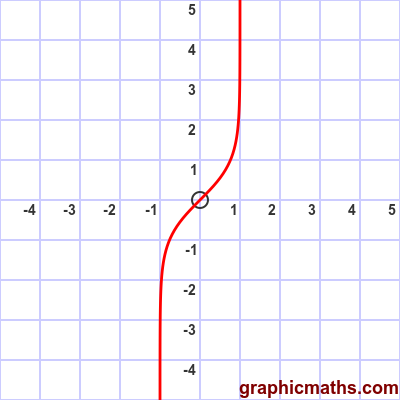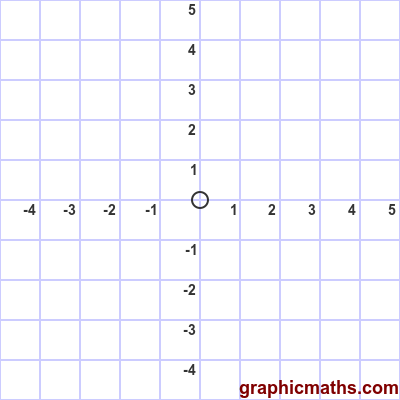# artanh function

By Martin McBride, 2020-02-03
Tags: artanh
Categories: hyperbolic functions

The artanh function is a hyperbolic function. It is the inverse of the tanh, and is also known as the inverse hyperbolic tangent function.

Why is it called the artanh rather than the arctanh? See here.

## Equation and graph

The artanh function is defined as the inverse of tanh, ie if:

$$x = \tanh y$$

then:

$$y = \operatorname{artanh} x$$

Here is a graph of the function:The function is only valid for -1 < x < 1.

## artanh as inverse of tanh

This animation illustrates the relationship between the tanh function and the artanh function:The first, blue, curve is the tanh function.

The grey dashed line is the line $y=x$.

The second, red, curve is the artanh function. As with any inverse function, it is identical to the original function reflected in the line $y=x$.

## Logarithm formula for artanh

There is a also a formula for finding artanh directly:

$$\operatorname{artanh} x =\frac12\ln\left(\frac{1+x}{1-x}\right)$$

Here is a proof of the logarithm formula for artanh. This follows similar lines to the proof for arsinh.

We will use:

$$u = \operatorname{artanh}{x}$$

The tanh of u will be x, because tanh is the inverse of artanh:

$$x = \tanh {u}$$

One form of the formula for tanh is:

$$\tanh{u} = \frac{e^{2u}-1}{e^{2u}+1}$$

This gives us:

$$x = \tanh{u} = \frac{e^{2u}-1}{e^{2u}+1}$$

Multiplying both sides by $(e^{2u}+1)$ gives:

$$({e^{2u}+1})x = e^{2u} - 1$$

Rearranging:

$$({e^{2u}+1})x - e^{2u} + 1 = 0$$

So:

$$e^{2u}(x - 1) + 1 + x = 0$$

$$e^{2u}(x - 1) = -(1 + x)$$

$$e^{2u} = -\frac{1 + x}{x - 1} = \frac{1 + x}{1-x}$$

Taking the log of both sides:

$$2u = \ln\left(\frac{1+x}{1-x}\right)$$

This gives the required result:

$$u = \operatorname{artanh} x =\frac12\ln\left(\frac{1+x}{1-x}\right)$$